In :
import itertools
import feedparser
import urllib
import io
import pandas
import skimage.segmentation
import networkx

/opt/local/Library/Frameworks/Python.framework/Versions/2.7/lib/python2.7/site-packages/pytz/__init__.py:35: UserWarning: Module argparse was already imported from /opt/local/Library/Frameworks/Python.framework/Versions/2.7/lib/python2.7/argparse.pyc, but /Users/fedorbaart/.virtualenvs/main/lib/python2.7/site-packages is being added to sys.path
from pkg_resources import resource_stream

In :
FEED='http://api.flickr.com/services/feeds/photos_public.gne?tags=beach;sand;waves'

In :
feed = feedparser.parse(FEED)

In :
photos = []
for entry in feed.entries:

In :
import IPython.display
IPython.display.Image(url=photos)

Out:In :
# Read the data as array
f.seek(0)

In :
# What do we have
plt.imshow(img)

Out:
<matplotlib.image.AxesImage at 0x100765410>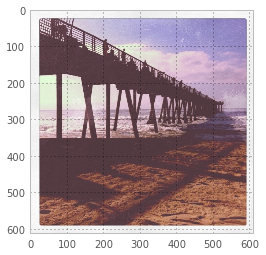In :
# Compute superpixels
slice=2 # Reduce because I don't have the fast version built
imgsliced = img[::slice,::slice]
superpixel = skimage.segmentation.slic(imgsliced, n_segments=200,ratio=20)

In :
# use a trick to show a segmented img
plt.imshow(superpixel % 11, cmap='Set3')

Out:
<matplotlib.image.AxesImage at 0x107d1b190>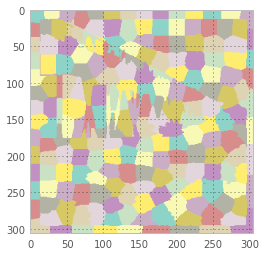In :
# Create a table of pixels
rows=[]
for (i,j), px in np.ndenumerate(superpixel):
row = [i,j,px]
row.extend(imgsliced[i,j])
rows.append(row)
df = pandas.DataFrame(data=rows, columns=["i", "j", "superpixel", "R", "G", "B"])

In :
# Compute superpixel properties
agg = df.groupby("superpixel").mean()
meaned = np.floor(agg.ix[superpixel.ravel()][["R", "G", "B"]].as_matrix()).astype('uint8')
meaned = meaned.reshape(imgsliced.shape)

In :
# Something went wrong with the means here...
# Triangulate to setup node connections
fig, ax = plt.subplots(figsize=(15,10))
ax.imshow(meaned)
# plot the mean location of each superpixel
ax.plot(agg.j, agg.i, 'k.')

Out:
[<matplotlib.lines.Line2D at 0x10979c450>]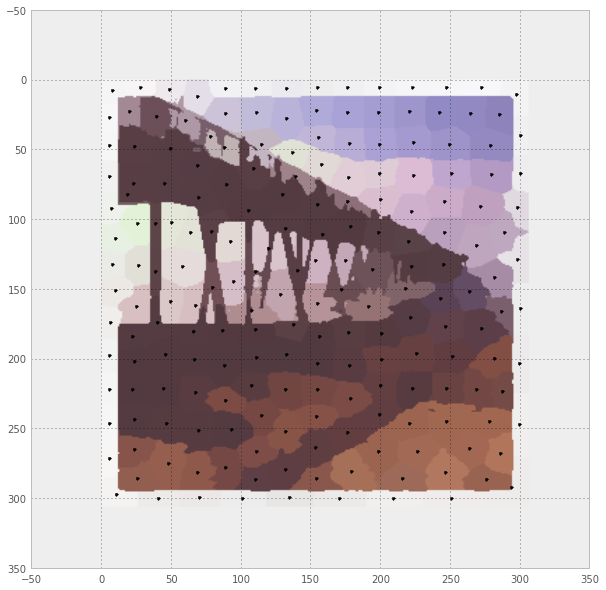In :
# Compute triangulation, can we reduce the number of connections?
tri = matplotlib.tri.triangulation.Triangulation(agg.j, agg.i)
matplotlib.tri.triplot(ax, tri)
# show the figure
fig

Out: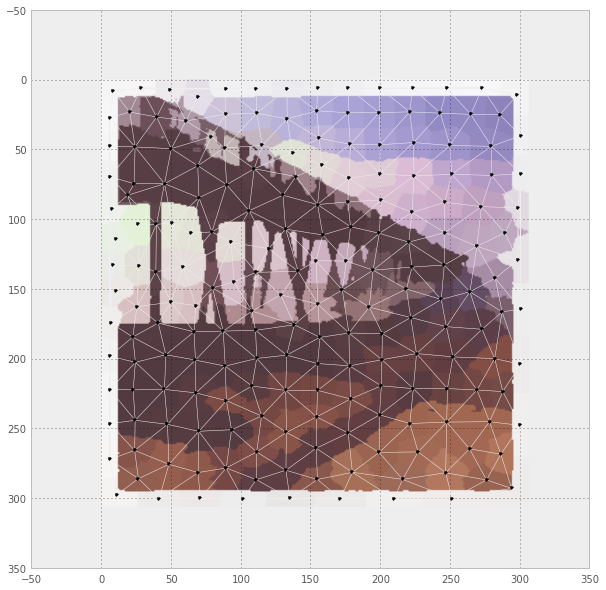In :
# Generate a graph from the triangular network
graph = networkx.Graph()

for (i, row) in agg.iterrows():

distances = np.sqrt(np.diff(tri.x[tri.edges],1)**2 + np.diff(tri.y[tri.edges],1)**2)
for (edge, distance) in zip(tri.edges, distances):

graphoriginal = graph.copy()

In :
# This should show up as a similar picture to the original node position
networkx.draw_spring(graph, weight="distance")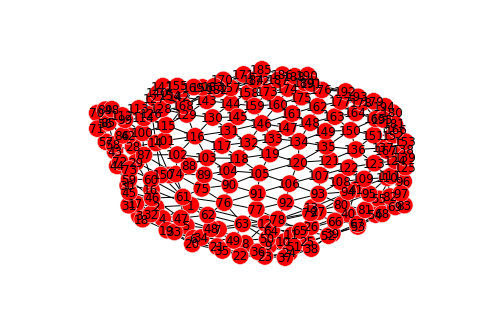In :
graph = graphoriginal.copy()

for node in graph.nodes():
plt.plot(agg.ix[node]['j'], agg.ix[node]['i'], 'k.')
for edge in graph.edges():
plt.plot(agg.ix[np.array(edge)]['j'], agg.ix[np.array(edge)]['i'], 'b-')

edges = sorted(graph.edges(data=True), key=lambda x:x['distance'], reverse=True)
i = 0.1
for edge in edges:
# can we toss this one out?
if len(graph.edge[edge]) >4 and len(graph.edge[edge]) >4:
plt.plot(agg.ix[np.array(edge[:2])]['j'], agg.ix[np.array(edge[:2])]['i'], linewidth=1/float(i), color='red')
i += 0.005
graph.remove_edge(edge, edge)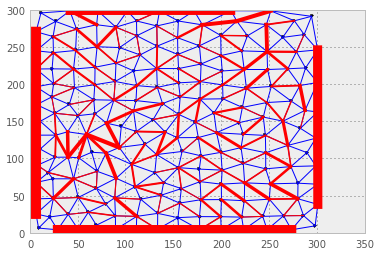In :
for node in graph.nodes():
plt.plot(agg.ix[node]['j'], agg.ix[node]['i'], 'k.')
for edge in graph.edges():
plt.plot(agg.ix[np.array(edge)]['j'], agg.ix[np.array(edge)]['i'], 'b-')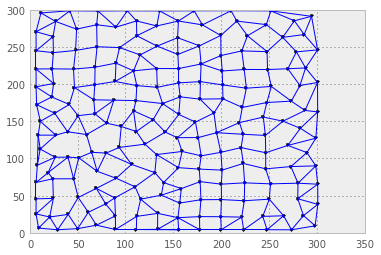In :
# Compute the number of rows and columns
N = len(agg)
# pixels per superpixel
R = float(imgsliced.shape)/float(imgsliced.shape)
# average height
nrow = int(round((N/R)**0.5))
ncol = int(round((N*R)**0.5))

# Pixelsize, approximately
S = np.sqrt((imgsliced.shape*imgsliced.shape)/float(N))
S, imgsliced.shape/S, imgsliced.shape/S

assert nrow*ncol == N, "{} != {}".format(nrow*ncol, N)

In :
# oh, it's just....
rows = superpixel % nrow
cols = superpixel // nrow
(cols*nrow  + rows == superpixel).all()

Out:
True
In :
df['m'] = rows.ravel()
df['n'] = cols.ravel()

In :
fig, ax = plt.subplots(figsize=(15,10))
ax.imshow(meaned)
# plot the mean location of each superpixel
ax.plot(agg.j, agg.i, 'k.')
for i in range(nrow):
for j in range(ncol):
node0 = j*nrow + i
node1 = j*nrow + i+1
node2 = (j+1)*nrow + i
edge1 = np.array([node0, node1])
edge2 = np.array([node0, node2])
if i<(nrow-1):
ax.plot(agg.ix[edge1]['j'], agg.ix[edge1]['i'], 'k-')
if j<(ncol-1):
ax.plot(agg.ix[edge2]['j'], agg.ix[edge2]['i'], 'k-')